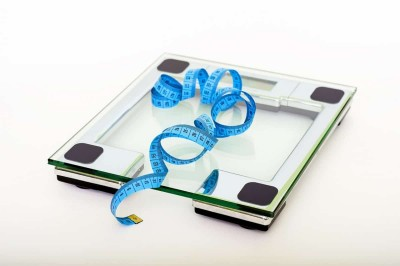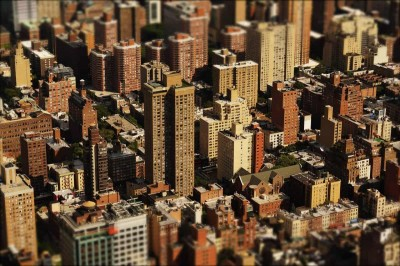# Does Standard Deviation Have Units? (5 Key Ideas To Know)

Standard deviation is a measure of spread that helps us to interpret data.  Together with the mean, standard deviation can tell us a lot about the data, and the units can also help us to understand the data.

So, does standard deviation have units?  Standard deviation has units that are the same as the units for the data values. Standard deviation can have square units if the data values have square units (for example, an area in square feet). Variance (standard deviation squared) has units that are the square of the units for the data values.

Of course, standard deviation will always have the same units as the mean, since both are measured in the units for the data values.

In this article, we’ll talk about the units for standard deviation and how they are determined.  We’ll also compare units for standard deviation, mean, variance, and the data values themselves.

Let’s get started.

## Does Standard Deviation Have Units?

Standard deviation has units.  No matter what quantity we are measuring in our data (height, weight, length, width, time, day length, etc.), the calculated standard deviation will have units.

The units can be linear (such as for lengths), squared (such as for areas), or cubed (such as for volumes). They can also be rates (such as for speeds).The units for standard deviation can be lengths, areas, volumes, and rates (such as the speed for a car).

### What Is Standard Deviation Measured In?

Standard deviation is measured in the same units as the data points that were used to calculate the standard deviation.

The units are the same for both sample standard deviation and population standard deviation (you can learn about the difference between them here).

#### Example 1: Standard Deviation Units For Height

If you have a set of data points that measure height in feet, then the standard deviation would also be given in terms of feet.If you are measuring height in inches, then the standard deviation will also have units of inches.

If you converted all of the data values to inches (multiply feet by 12 to get inches), then the standard deviation would be given in terms of inches.

If you convert the units for your data values, make sure to also report the standard deviation in the correct converted units!

#### Example 2: Standard Deviation Units For Area

If you have a set of data points that measure the area of property (lots) in acres, then the standard deviation would also be given in terms of acres.To measure the area of a lot, we would use square feet for our data values. In that case, the standard deviation would also be given in units of square feet.

If you converted all of the data values to square feet (multiply acres by 43,560 to get feet), then the standard deviation would be given in terms of square feet.

#### Example 3: Standard Deviation Units For Volume

If you have a set of data points that measure the volume of a pool in cubic feet, then the standard deviation would also be given in terms of cubic feet.The units of measurement for a pool could be in gallons. In that case, the standard deviation would also be given in gallons.

If you converted all of the data values to gallons (multiply cubic feet by 7.48 to get gallons), then the standard deviation would be given in terms of gallons.

#### Example 4: Standard Deviation Units For Speed (Or Velocity)

If you have a set of data points that measure the speed of an object (like a car) in meters per second, then the standard deviation would also be given in terms of meters per second.If we measure the speed of cars in meters per second, then the standard deviation of speeds would also be in given in units of meters per second.

If you converted all of the data values to miles per hour (multiply meters per second by 2.237 to get miles per hour), then the standard deviation would be given in terms of miles per hour.

#### Example 5: Standard Deviation Units For Acceleration

If you have a set of data points that measure the acceleration of an object (like a car) in meters per second squared (meters/second2), then the standard deviation would also be given in terms of meters per second squared (meters/second2).

If you converted all of the data values to miles per hour squared (multiply meters per second squared by 8053 to get miles per hour squared), then the standard deviation would be given in terms of miles per hour squared (miles/hour2).

### Is Standard Deviation In The Same Units As The Mean?

Standard deviation is in the same units as the mean for a given data set.  As a result, the units “match up” in a sense.

Since the units for mean and standard deviation are the same, it makes sense to talk about things like:

In the process of finding standard deviation, the units for the data points are squared (when we take squared differences).  However, we later take the square root of the sum of squared differences, which returns us to the original units.

The formula for standard deviation below suggests how the “squared” and “square root” (radical) cancel each other out.

You can also see how this works in the steps below.

To find the sample standard deviation, take the following steps:

• 1. Calculate the mean of the sample (add up all the values and divide by the number of values).
• 2. Calculate the difference between the sample mean and each data point.
• 3. Square the differences from Step 2.
• 4. Sum the squared differences from Step 3.
• 5. Divide the sum from Step 4 by n – 1 (the sample size minus one).
• 6. Take the square root of the quotient from Step 5.

To find the population standard deviation, the process is very similar to the one we used for finding samples standard deviation.  Here are the steps:

• 1. Calculate the mean of the sample (add up all the values and divide by the number of values).
• 2. Calculate the difference between the sample mean and each data point.
• 3. Square the differences from Step 2.
• 4. Sum the squared differences from Step 3.
• 5. Divide the sum from Step 4 by N (the population size).
• 6. Take the square root of the quotient from Step 5.

### Is Standard Deviation Units Squared?

The units for standard deviation are only squared when the units for mean (and the original data values) are also squared.

For example, if your data points are measurements for the area of lots in a city, then the units will be in square feet.  Since the data points have square units, the mean and standard deviation will also have square units.The area of a lot in a city would be measured in square feet, and so the standard deviation is also given in units of square feet.

### Does Variance Have Units?

Variance has units that are the square of the units for standard deviation.  This applies to both “pure” units (like feet or pounds) and also rates (miles per hour) or areas (square feet).

The table below gives some examples of how the units for variance compare to the units for standard deviation.

[table]

#### Example 1: Variance Units For Height

If you have a set of data points that measure height in feet, then the variance would be given in terms of feet squared (square feet).

If you converted all of the data values to square inches (multiply square feet by 122 = 144 to get square inches), then the variance would be given in terms of square inches.

If you convert the units for your data values, make sure to also report the variance in the correct converted units!

#### Example 2: Variance Units For Area

If you have a set of data points that measure the area of a garden in square yards (yard2), then the variance would be given in terms of yard4.

If you converted all of the data values to square feet (multiply square yards by 32 = 9 to get square feet), then the variance would be given in terms of feet4.

#### Example 3: Variance Units For Volume

If you have a set of data points that measure the volume of a pool in cubic feet (feet3), then the variance would be given in terms of feet6.

If you converted all of the data values to gallons (multiply cubic feet by 7.48 to get gallons), then the variance would be given in terms of gallons3.

#### Example 4: Variance Units For Speed (Or Velocity)

If you have a set of data points that measure the speed of an object (like a car) in meters per second, then the variance would also be given in terms of meters squared per second squared (meters2/second2).

If you converted all of the data values to miles per hour (multiply meters per second by 2.237 to get miles per hour), then the variance would be given in terms of miles squared per hour squared (miles2/hour2).

#### Example 5: Variance Units For Acceleration

If you have a set of data points that measure the acceleration of an object (like a car) in meters per second squared (meters/second2), then the variance would be given in terms of meters squared per second to the fourth (meters2/second4).

If you converted all of the data values to miles per hour squared (multiply meters per second squared by 8053 to get miles per hour squared), then the variance would be given in terms of miles squared per hour to the fourth (miles2/hour4).

### Does Range Have Units?

Range does have units, and they are the same units as the data values (and the same units as mean and standard deviation).

For example, if you measure the weight of dogs in pounds, then the range would be given in pounds also.

If your measurements, in pounds, were {25, 27, 33, 34, 40, 40, 41, 42, 50, 100}, then the range would be 100 – 25 = 75 pounds.If we measure the weights of dogs in pounds, then the standard deviation would also be given in units of pounds.

## Conclusion

Now you know all about the units for standard deviation and how they relate to the units for mean, variance, range, and the data values themselves.

You can learn about the difference between standard deviation and standard error here.